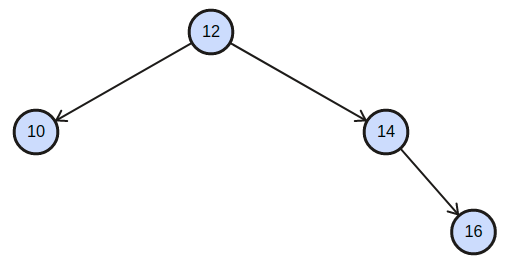New update is available. Click here to update.
Last Updated: 6 Nov, 2020
##### Sorted Linked List to Balanced BST
Moderate
Problem statement#### A Balanced BST is defined as a BST in which the height of two subtrees of every node differs no more than 1.

##### Input Format :
``````The first line of input contains an integer ‘T’ denoting the number of test cases to run. Then the test case follows.

The only line of each test case contains the elements of the singly linked list separated by a single space and terminated by -1. Hence, -1 would never be a list element.
``````
##### Output Format :
``````For each test case, print the nodes of the tree in the level order form separated by single spaces (Print -1 for NULL nodes). Refer to the below example for further clarification.

Print the output for every test case in a separate line.
``````
##### For Example
``````Consider the Binary Search Tree-
````````````The above BST will be printed as-
12 10 14 -1 -1 -1 16 -1 -1

Explanation :
Level 1 :
The root node of the tree is 12

Level 2 :
Left child of 12 = 10
Right child of 12 = 14

Level 3 :
Left child of 10 = null(-1)
Right child of 10 = null (-1)
Left child of 14 = null(-1)
Right child of 16 = 16

Level 4 :
Left child of 16 = null (-1)
Right child of 16 = null (-1)

The first not-null node (of the previous level) is treated as the parent of the first two nodes of the current level. The second not-null node (of the previous level) is treated as the parent node for the next two nodes of the current level and so on.

The output for each test case ends when all nodes at the last level are null (-1).
``````
##### Note :
``````You do not need to print anything, it has already been taken care of. Just implement the given function.
``````
##### Constraints :
``````1 <= T <= 100
0 <= N <= 1000
1 <= Data <= 10^9
Data != -1

Time Limit: 1sec
``````Approaches

## 01ApproachThe key observation here is that the middle node of the linked list would be the root of the BST. Therefore the nodes which lie to the left of the middle node will necessarily form the left subtree of the BST and which lies right to it will form the right subtree. So, we will devise a recursive solution based on this observation.

Algorithm

• We will keep the head pointer of the linked list as one of our parameters of the recursion function.
• We will find the pointer to the middle node of the linked list by the standard two pointers approach.
• The data of this node will be copied to the current root of the subtree.
• Let the previous pointer to the middle node be prev. We will set prev -> next = NULL.
• We will recurse on the left half of this linked list and by passing the head pointer as our parameter. This will necessarily become the left subtree of the current root node.
• We will recurse on the right half of the linked list by passing the middle -> next pointer as our parameter. This will necessarily become the right subtree of the current root node.